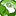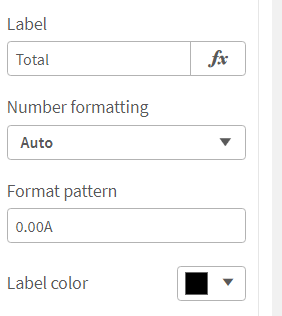# New to Qlik Sense

If you’re new to Qlik Sense, start with this Discussion Board and get up-to-speed quickly.

Announcements
cancel
Showing results for
Did you mean:Specialist

## Number formatting

HI All,

KPI is showing sum(sales ) as 1.58M, is it possible to convert it in number formatting to 1.6M.

In KPI I have used number formatting as Auto.

Labels (1)
• ### expression

1 Solution

Accepted SolutionsCreator III

Hi, if this sum(sales) number is static (or always be in millions), you can try something like this:

num( sum(sales) / 1000000 , '#.0M')

if it is dynamic, you can try something like this:

dual(num(sum(Sales)/pow(10,3*floor(log10(sum(Sales))/3)),'#.0'&pick(floor(log10(sum(Sales))/3),'k','M','B')),sum(Sales))

but change Number formatting to 'Measure expression' with this.

4 RepliesCreator III

Hi, if this sum(sales) number is static (or always be in millions), you can try something like this:

num( sum(sales) / 1000000 , '#.0M')

if it is dynamic, you can try something like this:

dual(num(sum(Sales)/pow(10,3*floor(log10(sum(Sales))/3)),'#.0'&pick(floor(log10(sum(Sales))/3),'k','M','B')),sum(Sales))

but change Number formatting to 'Measure expression' with this.Specialist II

Hi @Qlik1_User1 ,

Change the below format patter property as per your requirement.Regards,
Abhijit
keep Qliking...Specialist
Author

I am not getting this format option (I am looking for this in KPI)Specialist
Author

@justISO but sum(sales ) less than 1000 is getting missed by this expression.Tags